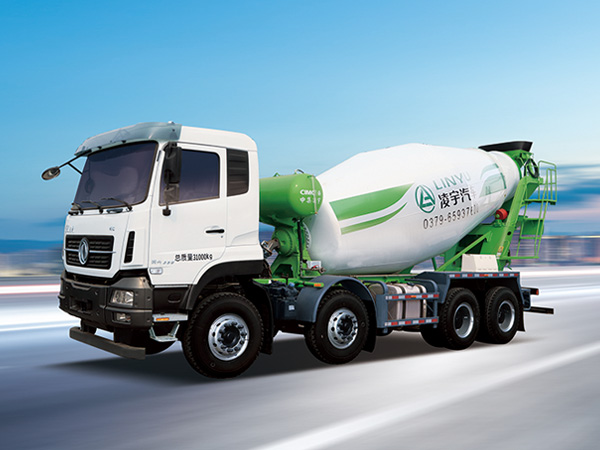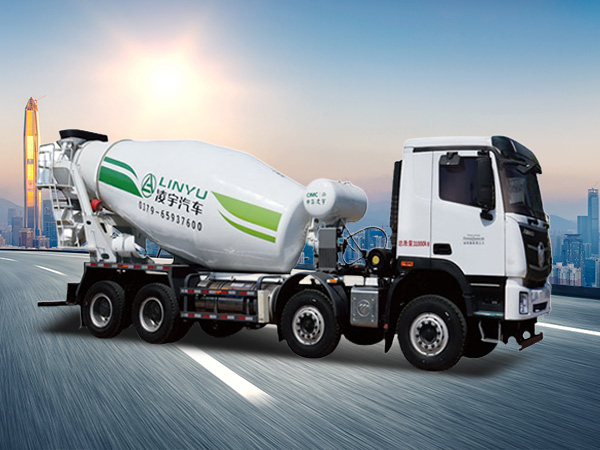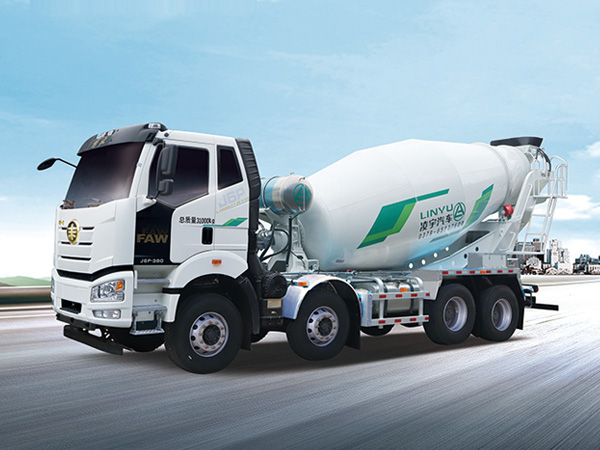2023-03-07

# How to calculate the capacity of concrete mixer truck

Concrete mixer truck capacity can vary depending on the manufacturer and model of the truck. However, typical capacities range from 3 to 8 cubic yards, with some larger models having capacities up to 12 cubic yards.

A cubic yard is a unit of volume that is equivalent to 27 cubic feet or 0.76 cubic meters. Therefore, a concrete mixer truck with a capacity of 3 cubic yards can carry up to 81 cubic feet or 2.28 cubic meters of concrete, while a truck with a capacity of 8 cubic yards can carry up to 216 cubic feet or 6.1 cubic meters of concrete.

The capacity of a concrete mixer truck can be calculated by multiplying the truck's volume by the material density. Here are the steps to calculate the capacity of a concrete mixer truck:Determine the mixer tank volume. This is the volume of the cylindrical mixer tank and is calculated by multiplying the tank radius by the tank height. For example, if the tank radius is 3 feet and the height is 10 feet, then the mixer tank volume is (3 feet)² x 3.14 x 10 feet = 282.6 cubic feet.

Convert the mixer tank volume to cubic yards. Since a cubic yard is equivalent to 27 cubic feet, divide the mixer tank volume by 27 to get the capacity of the mixer in cubic yards. Continuing with the example above, (282.6 cubic feet) / 27 = 10.47 cubic yards.

Determine the concrete density. Concrete density can vary depending on the mix design, but a typical density for normal weight concrete is 150 pounds per cubic foot or 2,400 kg per cubic meter.Multiply the mixer capacity in cubic yards by the concrete density in pounds per cubic foot (or kilograms per cubic meter) to find the weight capacity of the mixer in pounds (or kilograms). For example, if the mixer capacity is 10.47 cubic yards and the concrete density is 150 pounds per cubic foot, then the mixer capacity in pounds is 10.47 x 150 x 27 = 44,385 pounds.

By following these steps, you can calculate the capacity of a concrete mixer truck in either cubic yards or pounds (or cubic meters or kilograms). This information can be helpful in determining the appropriate size and capacity of a concrete mixer truck for a particular job or project.It's important to note that the capacity of a concrete mixer truck can also be affected by factors such as the thickness and consistency of the concrete being mixed, as well as the distance that the concrete needs to be transported. In some cases, larger trucks may be required to transport larger volumes of concrete over longer distances.

Concrete mixer trucks are commonly used in the construction industry for transporting and mixing concrete on job sites. The size and capacity of the truck can vary depending on the specific needs of the project and the amount of concrete required.

*
*
*
Send Request
News
CONTACT

Address:Zhangheng Street corner Guanlin Road, Science and Technology Park, Luolong District, Luoyang City, Henan Province

E-mail:inquiry@lingyu.com

Phone:0086-379-65937678